# Advanced Triangle Constructions

Go back to  'Triangles-Quadrilaterals'

The constructions of triangles, given different sets of its elements (angles or sides), was more or less straightforward. Now, we consider some more advanced constructions, where instead of elements directly, information about the triangle is provided indirectly.

Construction. Construct a triangle, given its base, one of the base angles, and the sum of the other two sides.

We are going to construct $$\Delta ABC$$, in which the base BC, base angle $$\angle B$$, and AB + AC are given.

Step 1: Draw the base BC, and through B, draw a ray BX inclined at the angle specified: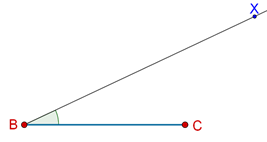Step 2: On BX, cut off BD equal to AB + AC: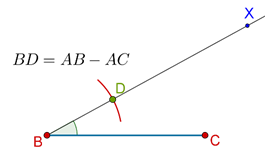Step 3: Join CD, and draw a ray CY such that $$\angle DCY$$= $$\angle BDC$$. Let that ray intersect the ray BX at A: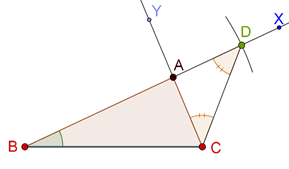$$\Delta ABC$$ is the required triangle. This is because in $$\Delta ACD$$, we will have AC = AD (due to our construction such that the opposite angles $$\angle DCY$$and $$\angle BDC$$are equal). Thus,

AB + AD = AB + AC

Alternatively, after Step 2, you could have drawn the perpendicular bisector of CD. The point of intersection of this perpendicular bisector with ray BX would also have given you point A: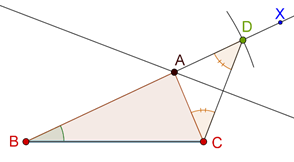This is because since A lies on the perpendicular bisector of CD, we will have AC = AD, and again:

AB + AD = AB + AC

Construction. Construct a triangle, given its base, a base angle and the difference of the other two sides.

We will construct $$\Delta ABC$$, given BC, $$\angle B$$, and the difference between AB and AC. Note that the difference between AB and AC does not specify which of the two will be larger. For example, if you are given that the difference between x and y is 3, there are two cases: x – y = 3, or y – x = 3. Therefore, we will have to consider two cases, one in which AB is larger, and one in which AC is larger.

### Case 1: AB > AC

The steps of the construction will be as follows:

Step 1: Draw BC, and draw a ray BX through B which is inclined to BC at the given angle value: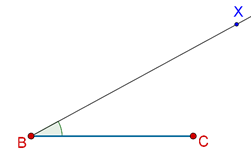Step 2: On BX, cut off BD equal to AB – AC:Step 3: Join CD and draw the perpendicular bisector of CD. Let it intersect ray BX at A: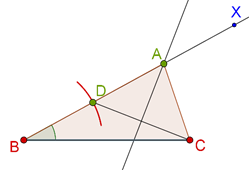$$\Delta ABC$$is the required triangle, because AC = AD (why?), and thus,

BD = AB – AD = AB – AC

### Case 2: AC > AB

The first step is the same (drawing BC and ray BX inclined to BC at the specified angle, but now, extend BX on the opposite side as well: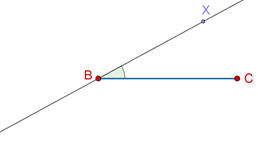Cut off BD = AC – AB on this extended ray: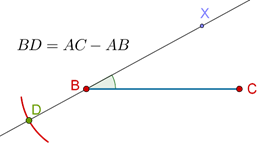Step 3: Join CD. Draw the perpendicular bisector of CD, and let it intersect ray BX at A: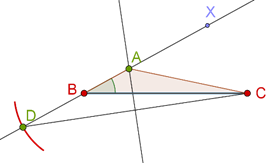$$\Delta ABC$$is the required triangle. This is because AC = AD (why?) and:

BD = AD – AB = AC – AB

Construction. Construct a triangle, given its perimeter and two base angles.

We will construct $$\Delta ABC$$, given $$\angle B$$, $$\angle C$$ and AB + BC + CA.

Step 1: Construct a segment PQ = AB + BC + CA, and draw rays PX and QY, inclined to PQ at $$\angle B$$ and $$\angle C$$ respectively.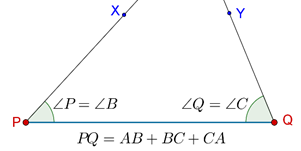Step 2: Draw the angle bisectors of $$\angle XPQ$$and $$\angle YQP$$, and denote their point of intersection by A: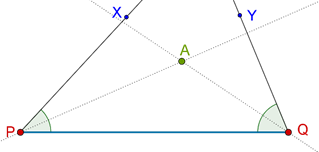Step 3: Draw the perpendicular bisectors of AP and AQ, and let them intersect PQ at B and C respectively: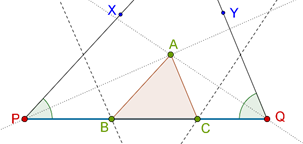$$\Delta ABC$$ is the required triangle. Let us see how:

1. Since B lies on the perpendicular bisector of AP, we have AB = PB. Similarly, AC = CQ, which means that AB + BC + CA = PB + BC + CQ = PQ. This is consistent with our earlier construction.

2. Since $$\Delta ABP$$ is isosceles, we have $$\angle BPA$$= $$\angle BAP$$. Thus, $$\angle ABC$$= $$\angle BPA$$+ $$\angle BAP$$= 2$$\angle BPA$$= $$\angle XPQ$$. Recall that we constructed angle $$\angle XPQ$$ equal to one of the given angles. Thus, $$\angle ABC$$ turns out to have the same value.

3. Similarly, $$\angle ACB$$ will turn out to be the same as $$\angle YQP$$, the other angle we were originally given.

Our entire construction is consistent and therefore correct. You are urged to re-read this discussion until you are absolutely clear about all the steps involved in it.

Example 1: Construct a $$\Delta ABC$$ such that AB = 4 cm, $$\angle A$$ = 600 and the sum of AC and BC is 11 cm.

Solution: The various steps of the construction are outlined below:

1. Draw AB = 4 cm, and ray AX such that $$\angle XAB$$= 600: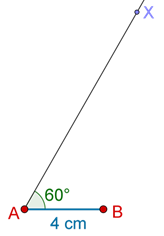Now, cut off AD = 11 cm on AX, join BD and draw the perpendicular bisector of BD. Let it intersect AX at C: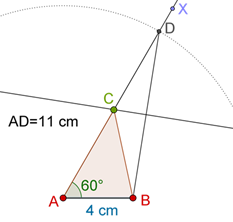$$\Delta ABC$$ is the required triangle. Justify this construction without referring to the earlier proof.

Example 2: Construct a $$\Delta ABC$$ such that $$\angle B$$ = 600, $$\angle C$$ = 450 and the perimeter of the triangle is 13 cm.

Solution: The relevant steps are outlined below:

1. Draw a segment PQ = 13 cm. Draw rays PX and QY such that $$\angle XPQ$$= 600 and $$\angle YQP$$= 450: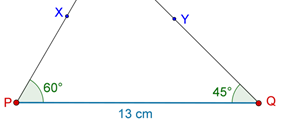Draw the angle bisectors of these two angles, and denote their point of intersection by A: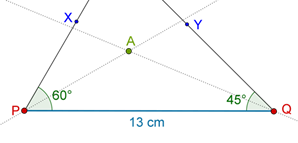Draw the perpendicular bisectors of AP and AQ, and denote their points of intersection with PQ by B and C: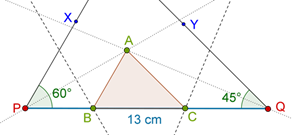$$\Delta ABC$$ is the required triangle. Once again, you are urged to justify this construction without referring to the earlier discussion.